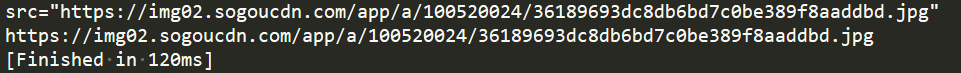### Python 正则表达式详解（建议收藏！）

match

. 匹配任意一个字符

\d 匹配数字

\D 匹配非数字

\s 匹配特殊字符，如空白，空格，tab等

\S 匹配非空白

\w 匹配单词、字符，如大小写字母，数字，_ 下划线

\W 匹配非单词字符

[ ] 匹配[ ]中列举的字符

* 出现0次或无数次

+ 至少出现一次

? 1次或则0次

{m}指定出现m次

{m,} 至少出现m次

{m,n} 指定从m-n次的范围

\$ 匹配结尾字符

^ 匹配开头字符

\b 匹配一个单词的边界

\B 匹配非单词边界

| 匹配左右任意一个表达式

(ab) 将括号中字符作为一个分组

search

findall

re.s

sub

split

提取图片地址

python中re模块提供了正则表达式的功能，常用的有四个方法(match、search、findall)都可以用于匹配字符串

# match

## 匹配字符串

re.match()必须从字符串开头匹配！match方法尝试从字符串的起始位置匹配一个模式，如果不是起始位置匹配成功的话，match()就返回none。主要参数如下：

``re.match(pattern, string)# pattern     匹配的正则表达式# string      要匹配的字符串``

``import rea = re.match('test','testasdtest')  print(a)                             #返回一个匹配对象print(a.group())                     #返回test，获取不到则报错print(a.span())           #返回匹配结果的位置，左闭右开区间print(re.match('test','atestasdtest'))  #返回None``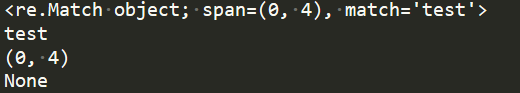## 单字符匹配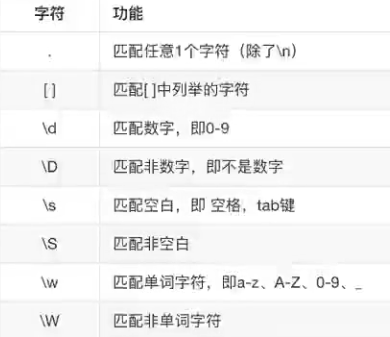### . 匹配任意一个字符

使用几个点号就代表几个字符

``import rea = re.match('..','testasdtest')  print(a.group())   #输出te                             b = re.match('ab.','testasdtest')  print(b) #返回none，因为表达式是以固定的ab开头然后跟上通配符. 所以必须要先匹配上ab才会往后进行匹配``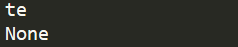### \d 匹配数字

一个\d代表一个数字。开头没匹配到，即使字符串其他部分包含需要匹配的内容，.match也会返回none

``import rea = re.match('\d\d','23es12testasdtest')  print(a)                               b = re.match('\d\d\d','23es12testasdtest')   print(b)   #要求匹配三个数字，匹配不到返回nonec = re.match('\d','es12testasdtest')   print(c)   #起始位置没有匹配成功，一样返回none``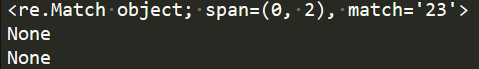### \D 匹配非数字

``import rea = re.match('\D','23es12testasdtest')  print(a)     #开头为数字所以返回none                          b = re.match('\D\D','*es12testasdtest')   print(b)   #返回*e``

### \s 匹配特殊字符，如空白，空格，tab等

``import reprint(re.match('\s',' 23es 12testasdtest'))   #匹配空格print(re.match('\s','   23es 12testasdtest')) #匹配tabprint(re.match('\s','\r23es 12testasdtest')) #匹配\r换行print(re.match('\s','23es 12testasdtest')) #返回none``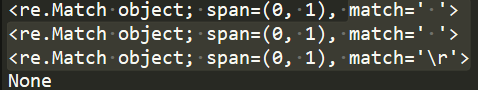### \S 匹配非空白

``import reprint(re.match('\S',' 23es 12testasdtest'))   #返回noneprint(re.match('\S','\r23es 12testasdtest'))   #noneprint(re.match('\S','23es 12testasdtest'))   ``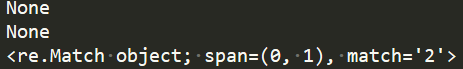### \w 匹配单词、字符，如大小写字母，数字，_ 下划线

``import reprint(re.match('\w','23es 12testasdtest'))   #返回noneprint(re.match('\w\w\w','aA_3es 12testasdtest'))   #返回noneprint(re.match('\w\w\w','\n12testasdtest'))   #返回none``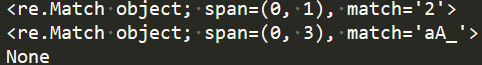### \W 匹配非单词字符

``import reprint(re.match('\W','23es 12testasdtest'))   #返回noneprint(re.match('\W',' 23es 12testasdtest'))   #匹配空格``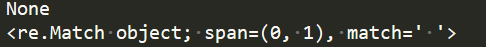### [ ] 匹配[ ]中列举的字符

``import reprint(re.match('12','232s12testasdtest'))  #因为开头的12没匹配上，所以直接返回noneprint(re.match('12','1232s12testasdtest')) #返回123``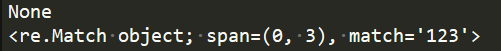[^2345] 不匹配2345中的任意一个

``import reprint(re.match('12[^234]','232s12testasdtest'))  #因为开头的12没匹配上，所以直接返回noneprint(re.match('12[^234]','1232s12testasdtest')) #返回noneprint(re.match('12[^234]','1252s12testasdtest')) #返回125``

[a-z3-5] 匹配a-z或者3-5中的字符

``import reprint(re.match('12[1-3a-c]','1232b12testasdtest'))  #123print(re.match('12[1-3a-c]','12b2b12testasdtest'))  #12bprint(re.match('12[1-3a-c]','12s2b12testasdtest'))  #返回none``

## 表示数量

像上面写的那些都是匹配单个字符，如果我们要匹配多个字符的话，只能重复写匹配符。这样显然是不人性化的，所以我们还需要学习表达数量的字符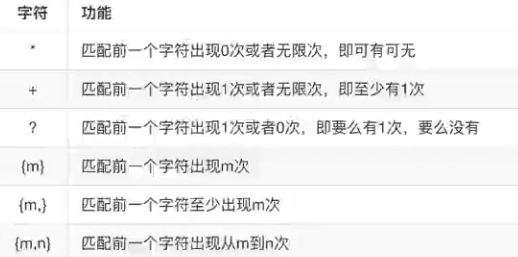### * 出现0次或无数次

``import rea = re.match('..','testasdtest')  print(a.group())   #输出te                             a = re.match('.*','testasdtest')  print(a.group())   #全部输出``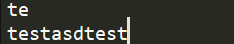``import reprint(re.match('a*','aatestasdtest')) #匹配跟随在字母a后面的所有a字符print(re.match('\d*','23aatestasdtest')) #匹配前面为数字的字符print(re.match('a\d*','ad23aatestasdtest')) #输出a, 因为*也可以代表0次``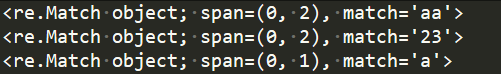### + 至少出现一次

``import reprint(re.match('a+','aaatestasdtest')) #匹配前面为字母a的字符，且a至少有1一个print(re.match('a+','atestasdtest'))   #aprint(re.match('a+','caaatestasdtest'))  #none``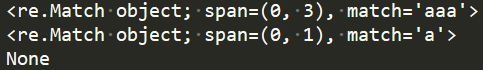### ? 1次或则0次

``import reprint(re.match('a?','abatestasdtest')) #匹配a出现0次或者1次数print(re.match('a?','batestasdtest'))  #输出空，因为a可以为0次print(re.match('a?','aaatestasdtest')) #a出现0次或者1次，输出1个a``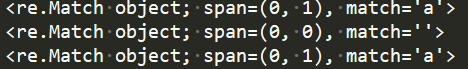### {m}指定出现m次

``import reprint(re.match('to{3}','toooooabatestasdtest')) #匹配t以及跟随在后面的三个oooprint(re.match('to{3}','tooabatestasdtest')) #只有两个0,返回none``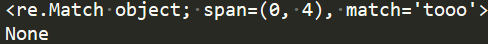### {m,} 至少出现m次

``import reprint(re.match('to{3,}','toooooabatestasdtest')) #匹配t以及跟随在后面的三个ooo至少出现3次print(re.match('to{3,}','tooabatestasdtest')) #只有两个0,返回none``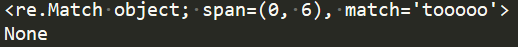### {m,n} 指定从m-n次的范围

``import reprint(re.match('to{3,4}','toooabatestasdtest')) #刚好有三个ooo，成功匹配print(re.match('to{3,4}','tooabatestasdtest'))  #只有两个o,返回noneprint(re.match('to{3,4}','toooooabatestasdtest')) #提取最多四个o``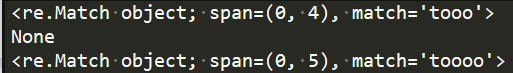## 匹配边界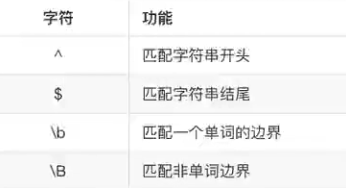### \$ 匹配结尾字符

``import reprint(re.match('.*d\$','2testaabcd')) #字符串必须以d结尾 print(re.match('.*c','2testaabcd'))  #字符串不是以c结尾，返回none``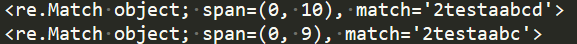### ^ 匹配开头字符

``import reprint(re.match('^2','2stoooabatestas')) #规定必须以2开头，否则none print(re.match('^2s','2stoooabatestas')) #必须以2s开头``

### \b 匹配一个单词的边界

\b：表示字母数字与非字母数字的边界，非字母数字与字母数字的边界。即下面ve的右边不能有字母和数字

``import reprint(re.match(r'.*ve\b','ve.2testaabcd'))  #因为在python中\代表转义，所以前面加上r消除转义print(re.match(r'.*ve\b','ve2testaabcd'))``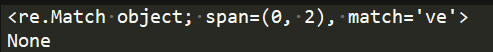### \B 匹配非单词边界

``import reprint(re.match(r'.*ve\B','2testaavebcdve'))  #ve的右边需要有字母或者数字 print(re.match(r'.*ve\B','2testaave3bcdve'))``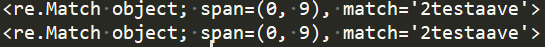## 匹配分组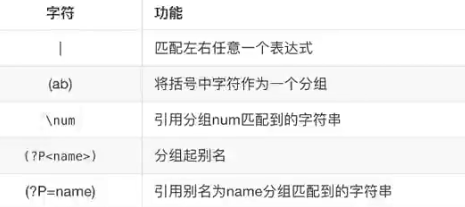### | 匹配左右任意一个表达式

``import reprint(re.match(r'\d[1-9]|\D[a-z]','2233'))  #匹配|两边任意一个表达式print(re.match(r'\d[1-9]|\D[a-z]','as'))  ``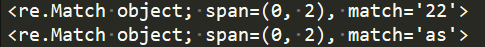### (ab) 将括号中字符作为一个分组

()中的内容会作为一个元组字符装在元组中

``import rea = re.match(r'<h1>(.*)<h1>','<h1>你好啊<h1>')print(a.group())    #输出匹配的字符print(a.groups())   #会将()中的内容会作为一个元组字符装在元组中print('`````````````')b = re.match(r'<h1>(.*)(<h1>)','<h1>你好啊<h1>')print(b.groups()) #有两括号就分为两个元组元素print(b.group(0))  #group中默认是0print(b.group(1))  #你好啊print(b.group(2))  #h1``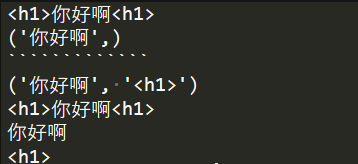# search

``import reprint(re.match(r'\d\d','123test123test'))print(re.search(r'\d\d','123test123test'))``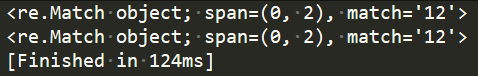# findall

``import reprint(re.search(r'test','123test123test'))print(re.findall(r'test','123test123test'))  #以列表的方式返回``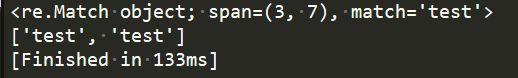## re.s

findall中另外一个属性re.S

• 如果不使用re.S参数，则只在每一行内进行匹配，如果一行没有，就换下一行重新开始。
• 而使用re.S参数以后，正则表达式会将这个字符串作为一个整体，在整体中进行匹配。

如下要寻找test.*123的数据，因为test和123在不同的行，如果没加re.s的话，他会在每一个进行匹配查找而不是将字符串作为一个整体进行查找

``import rea = """aaatestaa     aaaa123"""print(re.findall(r'test.*123',a))       print(re.findall(r'test.*123',a,re.S))``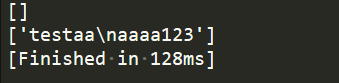# sub

sub(要替换的数据，替换成什么，要替换的数据所在的数据)

``import reprint(re.sub('php','python','php是世界上最好的语言——php'))  #输出 "python是世界上最好的语言——python"``

# split

``import res = "itcase,java:php-php3;html"print(re.split(r",",s))           #以,号进行分割print(re.split(r",|:|-|;",s))     #以,或者：或者-或者;进行分割print(re.split(r",|:|-|%",s))    #找不到的分隔符就忽略``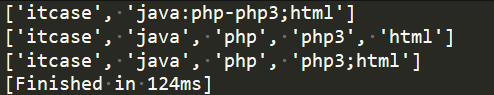# 贪婪与非贪婪

python里的数量词默认是贪婪的，总是尝试尽可能的匹配更多的字符。python中使用?号关闭贪婪模式

``import reprint(re.match(r"aa\d+","aa2323"))   #会尽可能多的去匹配\dprint(re.match(r"aa\d+?","aa2323"))  #尽可能少的去匹配\d``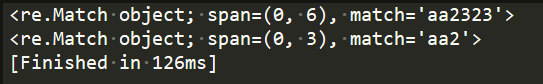``import res = "this is a number 234-235-22-423"# 1.贪婪模式resule = re.match(r"(.+)(\d+-\d+-\d+-\d)",s)   #我们本想数字和字母拆解成两个分组print(resule.groups())  #('this is a number 23', '4-235-22-4')但我们发现输出的结果中23的数字竟然被弄到前面去了 #因为+它会尽可能多的进行匹配，\d，只需要一个4就能满足，所以前面就尽可能多的匹配# 2.关闭贪婪模式#在数量词后面加上 ?，进入非贪婪模式，尽可能少的进行匹配result = re.match(r"(.+?)(\d+-\d+-\d+-\d)",s)print(result.groups())   #('this is a number ', '234-235-22-4')``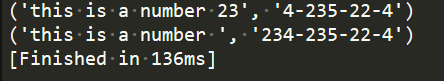# 案例

## 匹配手机号

``import reresult = re.match(r'1\d{9}','13111111111')print(result.group())   #匹配成功result = re.match(r'1\d{9}','12111111111')print(result)     #none，第二位为2result = re.match(r'1\d{9}','121111111112')print(result)     #none，有12位``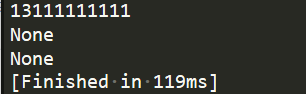## 提取网页源码中所有的文字

``s = """<div><p>岗位职责:</p><p>完成推荐算法、数据统计、接口、后台等服务器端相关工作</p><p><br></p><P>必备要求:</p><p>良好的自我驱动力和职业素养，工作积极主动、结果导向</p><p>&nbsp;<br></p><p>技术要求:</p><p>1、一年以上 Python开发经验，掌握面向对象分析和设计，了解设计模式</p><p>2、掌握HTTP协议，熟悉NVC、MVVM等概念以及相关wEB开发框架</p><p>3、掌握关系数据库开发设计，掌握SQL，熟练使用 MySQL/PostgresQL中的一种<br></p><p>4、掌握NoSQL、MQ，熟练使用对应技术解决方案</p><p>5、熟悉 Javascript/cSS/HTML5，JQuery,React.Vue.js</p><p>&nbsp;<br></p><p>加分项:</p><p>大数据，数理统计，机器学习，sklearn，高性能，大并发。</p></div>"""``

``result = re.sub(r'<.*?>|&nbsp','',s)  #print(result)``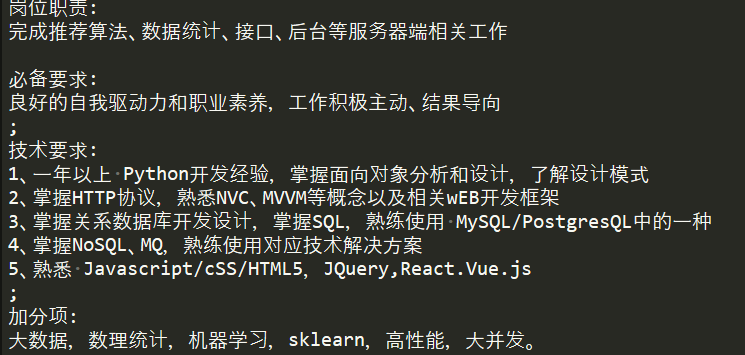如果关闭贪婪模式，<xx>中的内容会尽可能多的匹配，只要能够满足后面的>就行，然后<>xxx<>中xxx内容也替换掉了

## 提取图片地址

``import res = """<img data-original="https://img02.sogoucdn.com/app/a/100520024/36189693dc8db6bd7c0be389f8aaddbd.jpg" src="https://img02.sogoucdn.com/app/a/100520024/36189693dc8db6bd7c0be389f8aaddbd.jpg" width="250" height="375" .jpg>"""result1 = re.search(r"src=\"https.*.jpg\"",s)   print(result1.group()) result2 = re.search(r"src=\"(https.*.jpg)\"",s) #我只是想将网址提取出来，所以httpxx加括号，这样我就可以把它单独提取出来，src则不会出来print(result2.groups())``